# Representing Relations Epp section CS 202 Aaron Bloomfield

• Slides: 28
Download presentationRepresenting Relations Epp section ? ? ? CS 202 Aaron Bloomfield 1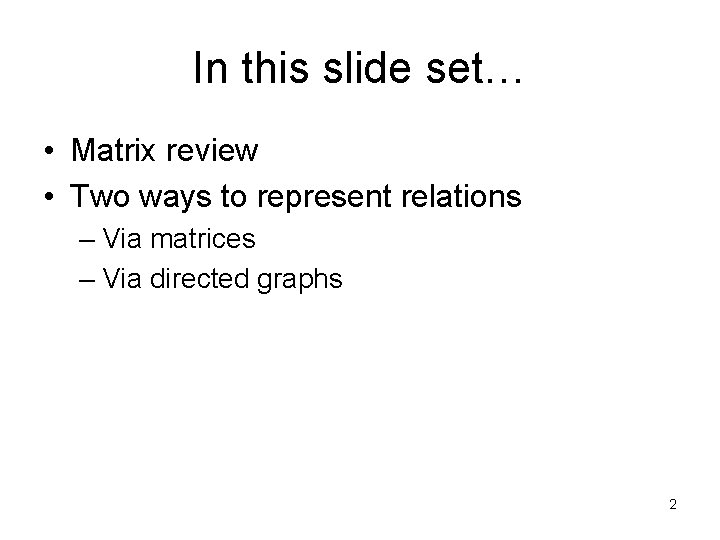In this slide set… • Matrix review • Two ways to represent relations – Via matrices – Via directed graphs 2Matrix review • We will only be dealing with zero-one matrices – Each element in the matrix is either a 0 or a 1 • These matrices will be used for Boolean operations – 1 is true, 0 is false 3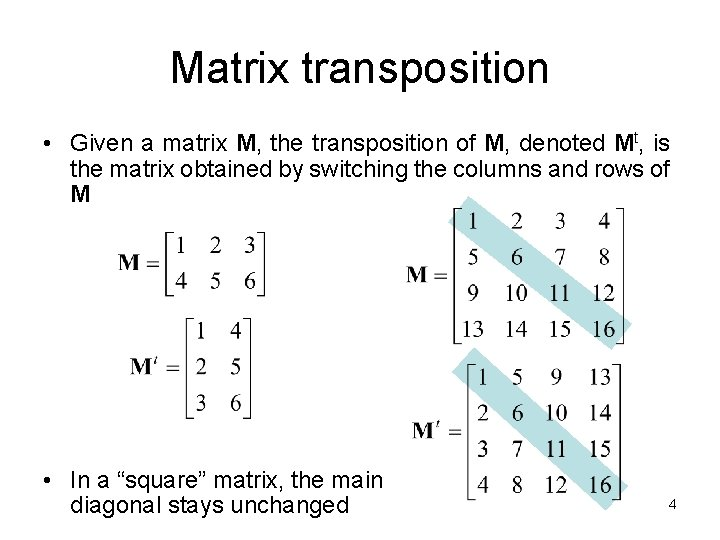Matrix transposition • Given a matrix M, the transposition of M, denoted Mt, is the matrix obtained by switching the columns and rows of M • In a “square” matrix, the main diagonal stays unchanged 4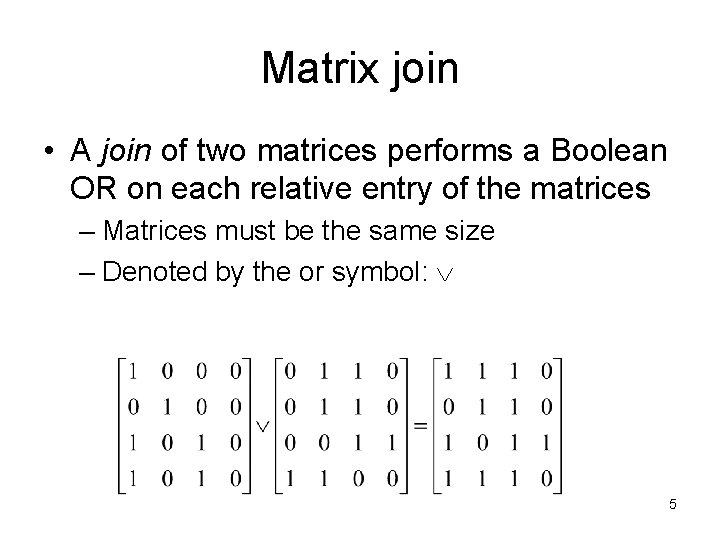Matrix join • A join of two matrices performs a Boolean OR on each relative entry of the matrices – Matrices must be the same size – Denoted by the or symbol: 5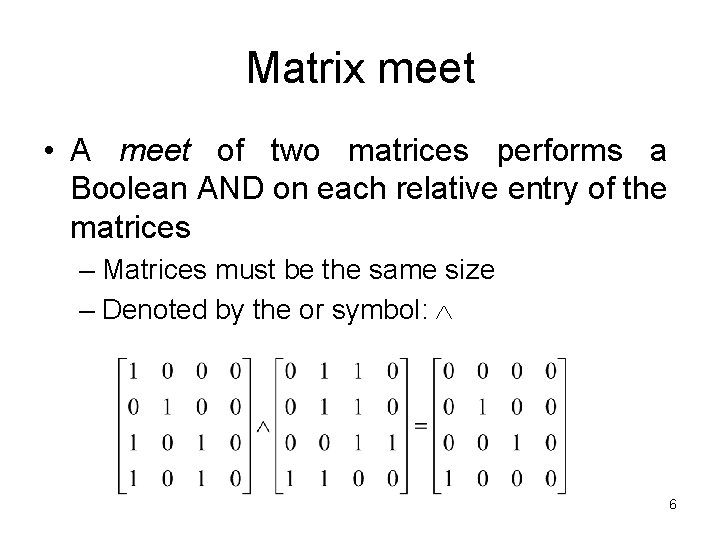Matrix meet • A meet of two matrices performs a Boolean AND on each relative entry of the matrices – Matrices must be the same size – Denoted by the or symbol: 6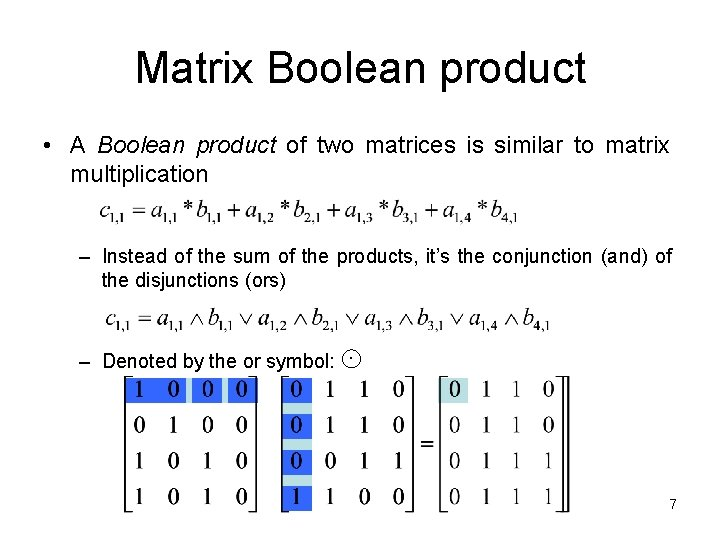Matrix Boolean product • A Boolean product of two matrices is similar to matrix multiplication – Instead of the sum of the products, it’s the conjunction (and) of the disjunctions (ors) – Denoted by the or symbol: 7Relations using matrices • List the elements of sets A and B in a particular order – Order doesn’t matter, but we’ll generally use ascending order • Create a matrix 8Relations using matrices • Consider the relation of who is enrolled in which class – Let A = { Alice, Bob, Claire, Dan } – Let B = { CS 101, CS 202 } – R = { (a, b) | person a is enrolled in course b } CS 101 CS 202 Alice Bob X X X Claire Dan 9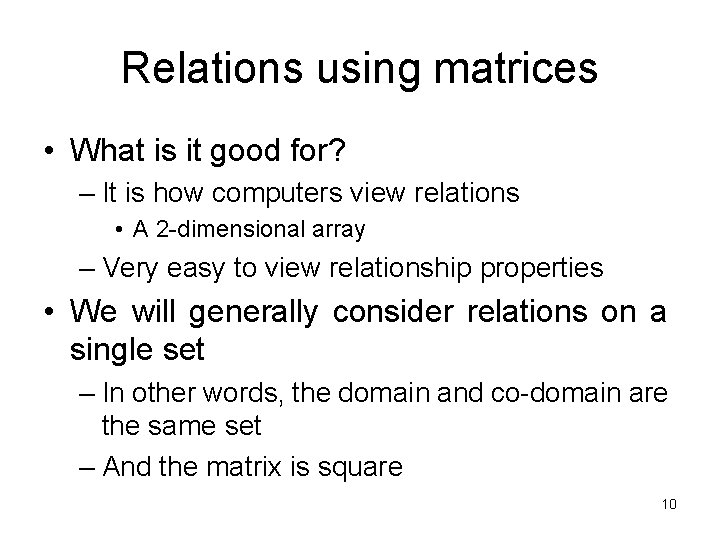Relations using matrices • What is it good for? – It is how computers view relations • A 2 -dimensional array – Very easy to view relationship properties • We will generally consider relations on a single set – In other words, the domain and co-domain are the same set – And the matrix is square 10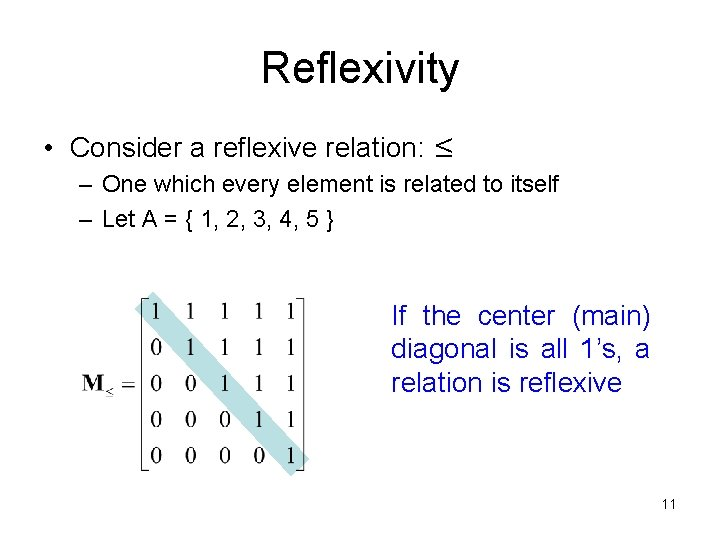Reflexivity • Consider a reflexive relation: ≤ – One which every element is related to itself – Let A = { 1, 2, 3, 4, 5 } If the center (main) diagonal is all 1’s, a relation is reflexive 11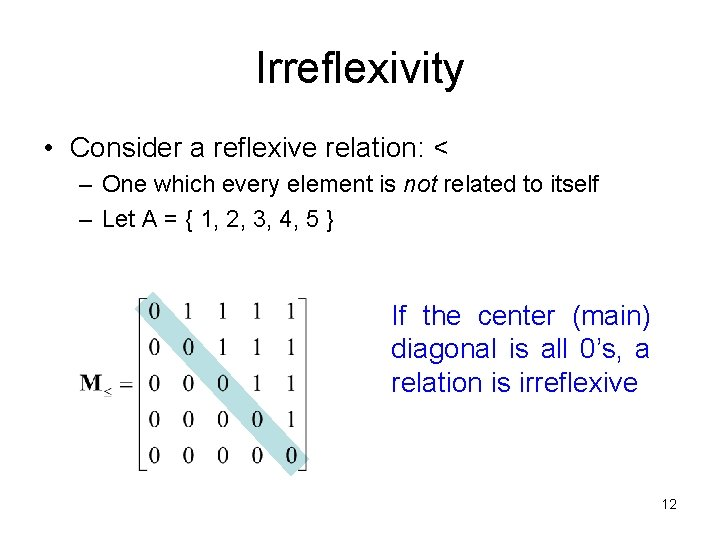Irreflexivity • Consider a reflexive relation: < – One which every element is not related to itself – Let A = { 1, 2, 3, 4, 5 } If the center (main) diagonal is all 0’s, a relation is irreflexive 12Symmetry • Consider an symmetric relation R – One which if a is related to b then b is related to a for all (a, b) – Let A = { 1, 2, 3, 4, 5 } • If, for every value, it is the equal to the value in its transposed position, then the relation is symmetric 13Asymmetry • Consider an asymmetric relation: < – One which if a is related to b then b is not related to a for all (a, b) • If, for every value and – Let A = { 1, 2, 3, 4, 5 } the value in its transposed position, if they are not both 1, then the relation is asymmetric • An asymmetric relation must also be irreflexive • Thus, the main 14 diagonal must be all 0’sAntisymmetry • Consider an antisymmetric relation: ≤ – One which if a is related to b then b is not related to a unless a=b for all (a, b) • If, for every value and – Let A = { 1, 2, 3, 4, 5 } the value in its transposed position, if they are not both 1, then the relation is antisymmetric • The center diagonal can have both 1’s and 0’s 15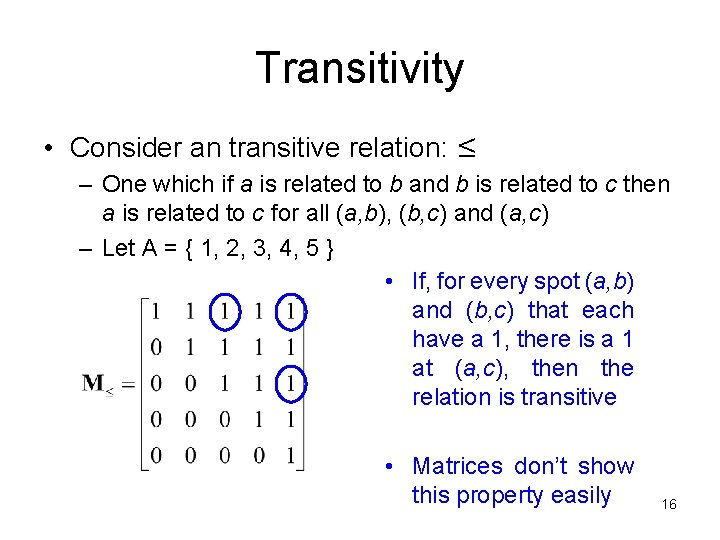Transitivity • Consider an transitive relation: ≤ – One which if a is related to b and b is related to c then a is related to c for all (a, b), (b, c) and (a, c) – Let A = { 1, 2, 3, 4, 5 } • If, for every spot (a, b) and (b, c) that each have a 1, there is a 1 at (a, c), then the relation is transitive • Matrices don’t show this property easily 16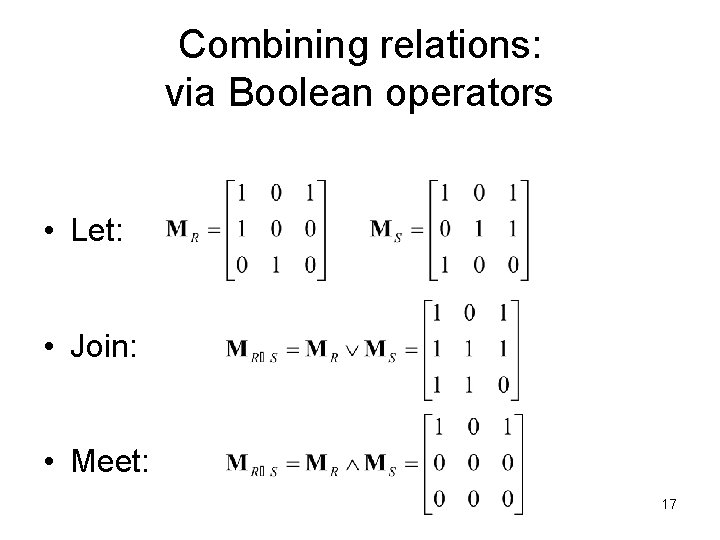Combining relations: via Boolean operators • Let: • Join: • Meet: 17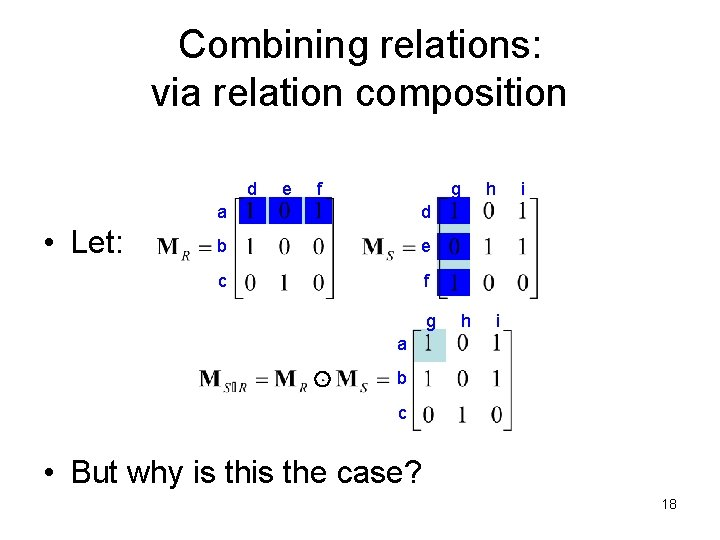Combining relations: via relation composition d • Let: e f g a d b e c f g h h i i a b c • But why is the case? 18Representing relations using directed graphs • A directed graph consists of: – A set V of vertices (or nodes) – A set E of edges (or arcs) – If (a, b) is in the relation, then there is an arrow from a to b • Will generally use relations on a single set • Consider our relation R = { (a, b) | a divides b } 1 1 • Old way: 2 2 3 3 4 4 1 2 3 4 19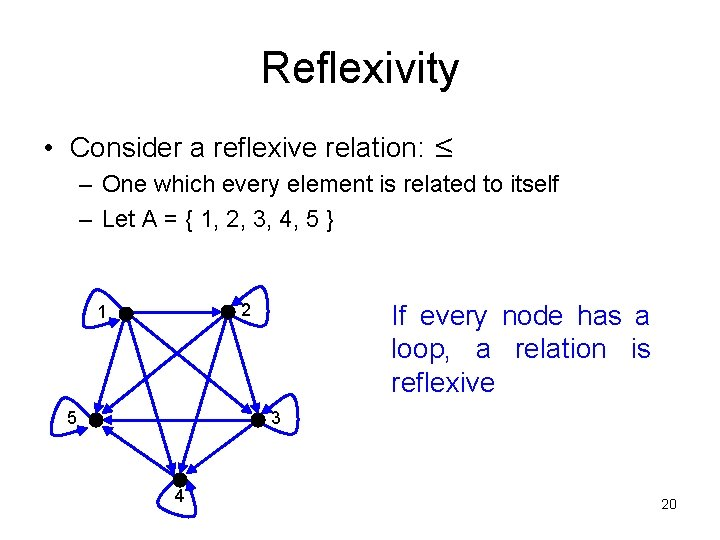Reflexivity • Consider a reflexive relation: ≤ – One which every element is related to itself – Let A = { 1, 2, 3, 4, 5 } If every node has a loop, a relation is reflexive 2 1 5 3 4 20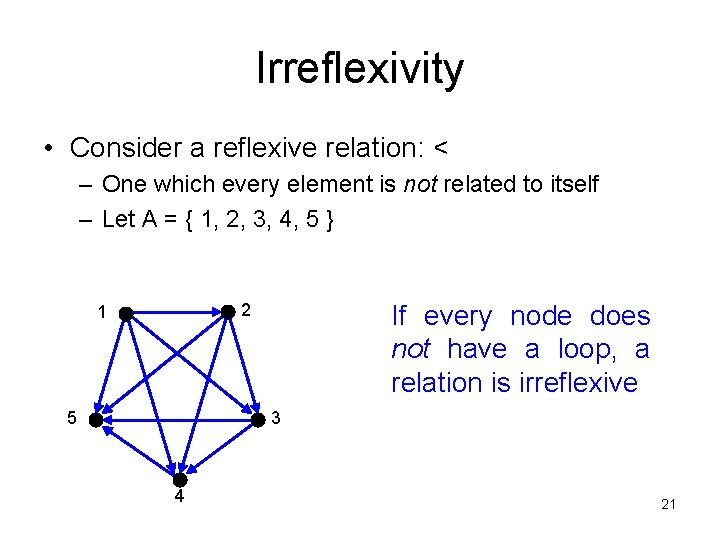Irreflexivity • Consider a reflexive relation: < – One which every element is not related to itself – Let A = { 1, 2, 3, 4, 5 } If every node does not have a loop, a relation is irreflexive 2 1 5 3 4 21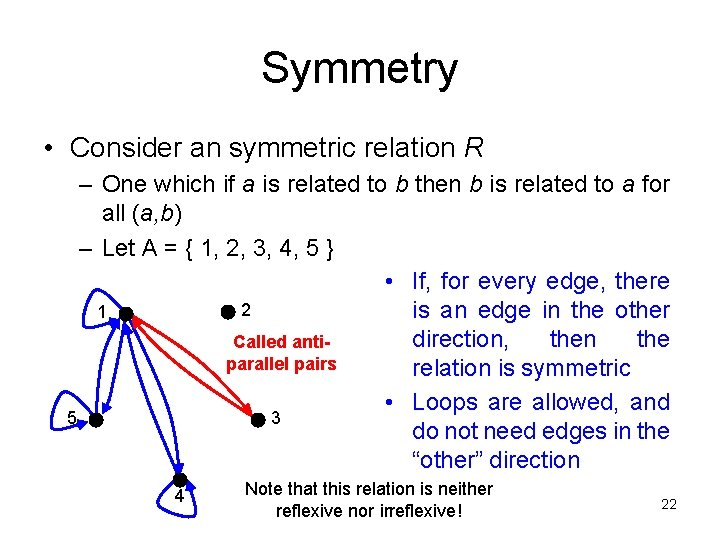Symmetry • Consider an symmetric relation R – One which if a is related to b then b is related to a for all (a, b) – Let A = { 1, 2, 3, 4, 5 } • If, for every edge, there 2 1 is an edge in the other direction, then the Called antiparallel pairs relation is symmetric • Loops are allowed, and 5 3 do not need edges in the “other” direction 4 Note that this relation is neither reflexive nor irreflexive! 22Asymmetry • Consider an asymmetric relation: < – One which if a is related to b then b is not related to a for all (a, b) – Let A = { 1, 2, 3, 4, 5 } • A digraph is asymmetric if: 2 1 1. If, for every edge, there is not an edge in the other direction, then the relation is asymmetric 5 3 2. Loops are not allowed in an asymmetric digraph (recall it must be irreflexive) 4 23Antisymmetry • Consider an antisymmetric relation: ≤ – One which if a is related to b then b is not related to a unless a=b for all (a, b) – Let A = { 1, 2, 3, 4, 5 } • If, for every edge, there is not an edge in the other 2 1 direction, then the relation is antisymmetric 5 3 4 • Loops are allowed in the digraph 24Transitivity • Consider an transitive relation: ≤ – One which if a is related to b and b is related to c then a is related to c for all (a, b), (b, c) and (a, c) – Let A = { 1, 2, 3, 4, 5 } • 2 1 5 3 4 A digraph is transitive if, for there is a edge from a to c when there is a edge from a to b and from b to c 25Applications of digraphs: Map. Quest • Not reflexive • Is irreflexive • Not symmetric • Not antisymmetric • Not transitive End • Not reflexive • Is irreflexive • Is symmetric • Not antisymmetric • Not transitive Start 26Sample questions Which of the graphs are reflexive, irreflexive, symmetric, antisymmetric, or transitive 23 24 25 26 27 28 Reflexive Irreflexive Y Y Symmetric Asymmetric Antisymmetric Transitive Y Y Y Y 27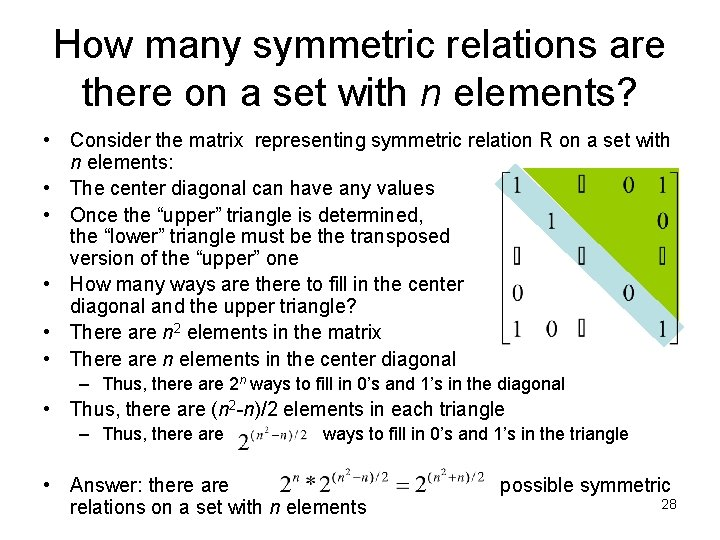How many symmetric relations are there on a set with n elements? • Consider the matrix representing symmetric relation R on a set with n elements: • The center diagonal can have any values • Once the “upper” triangle is determined, the “lower” triangle must be the transposed version of the “upper” one • How many ways are there to fill in the center diagonal and the upper triangle? • There are n 2 elements in the matrix • There are n elements in the center diagonal – Thus, there are 2 n ways to fill in 0’s and 1’s in the diagonal • Thus, there are (n 2 -n)/2 elements in each triangle – Thus, there are ways to fill in 0’s and 1’s in the triangle • Answer: there are relations on a set with n elements possible symmetric 28